# Chapter10 Revised

Broward County Schools
27 de Jan de 2010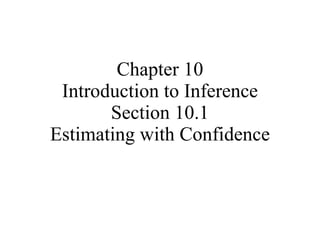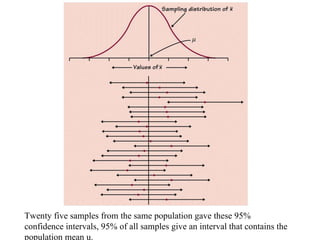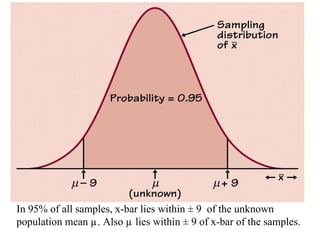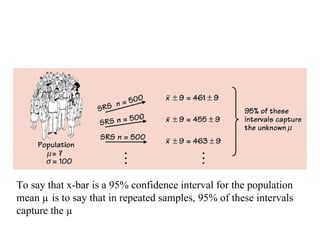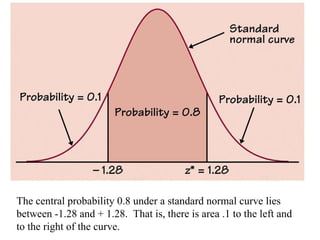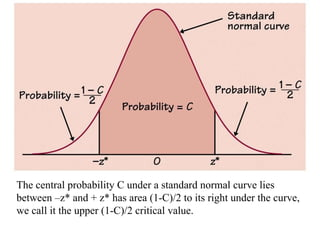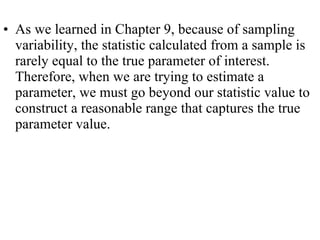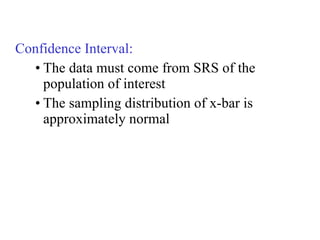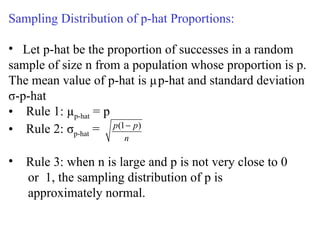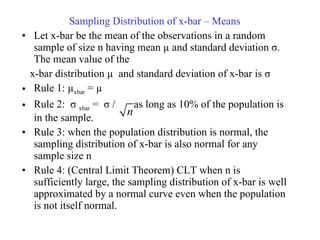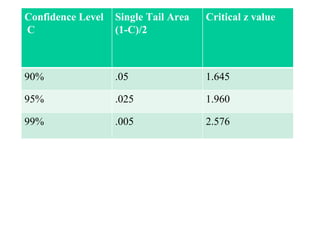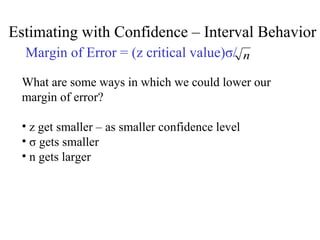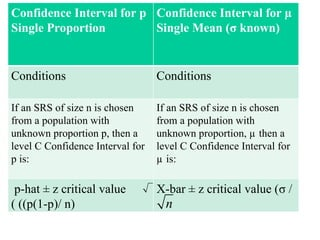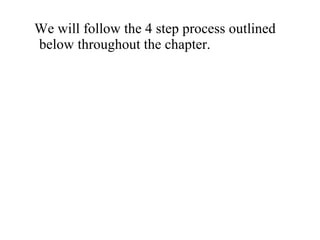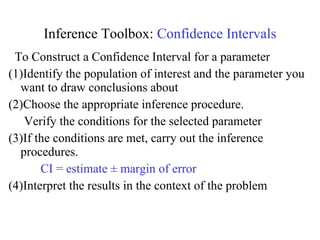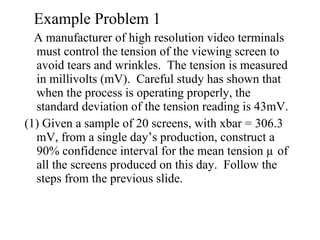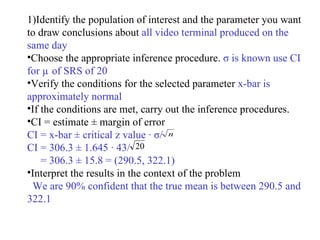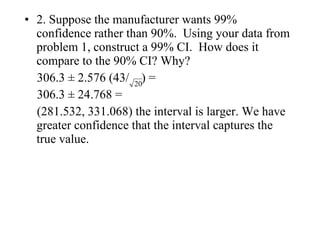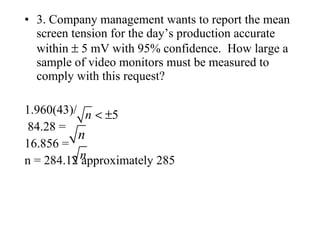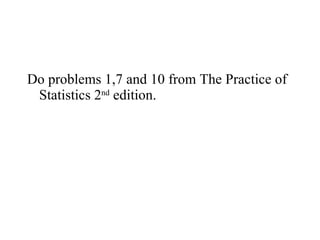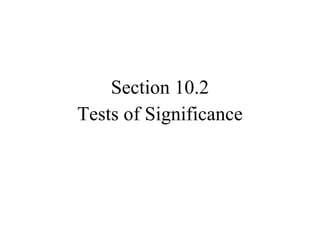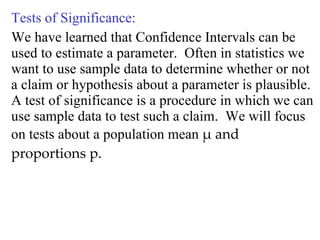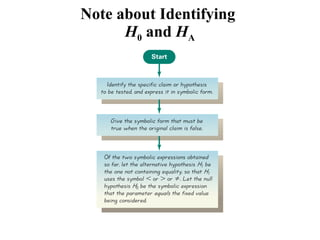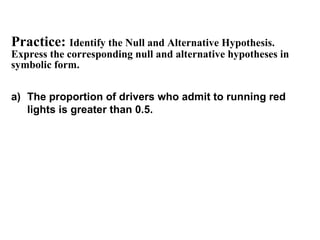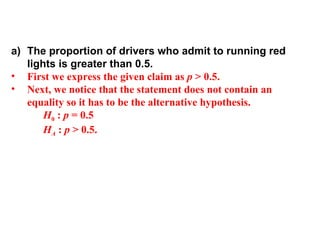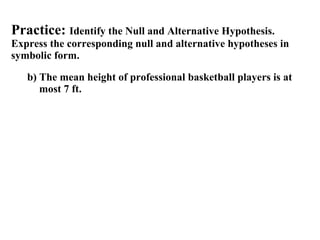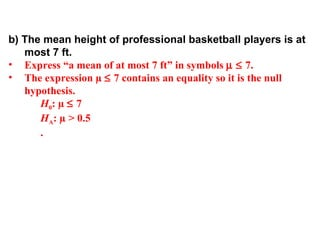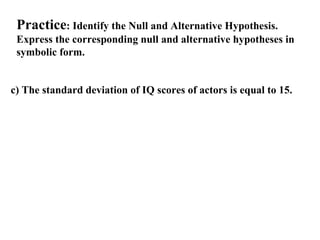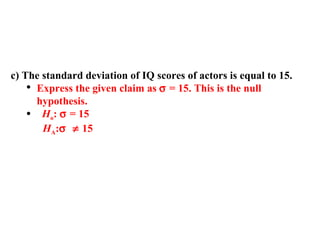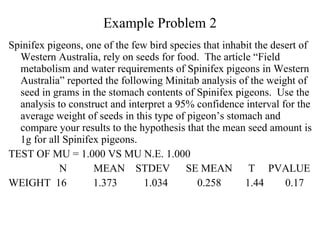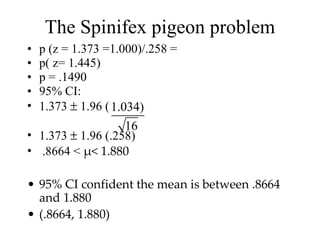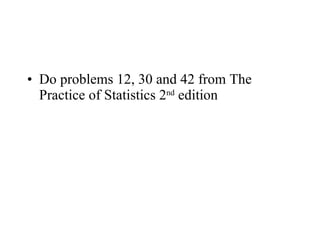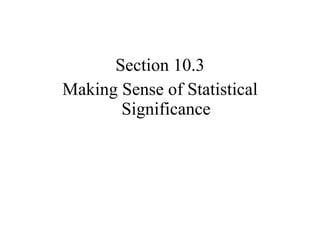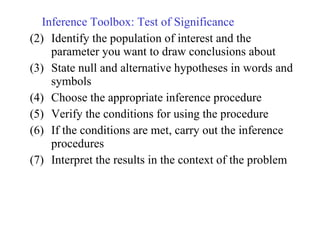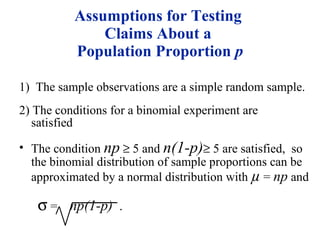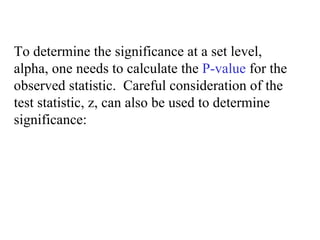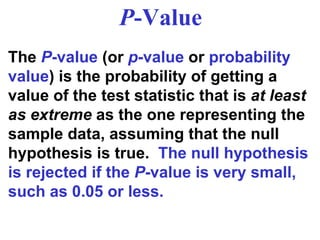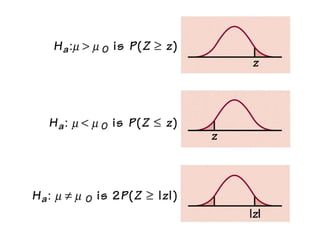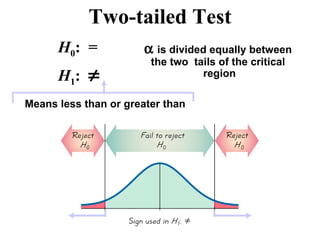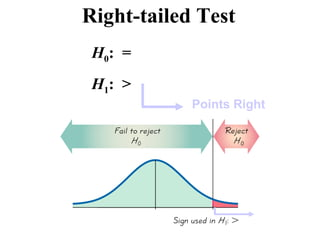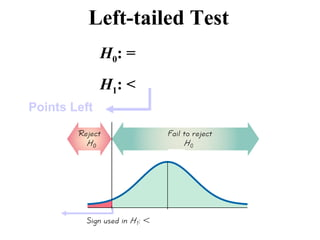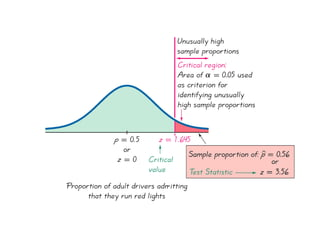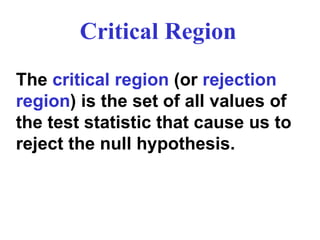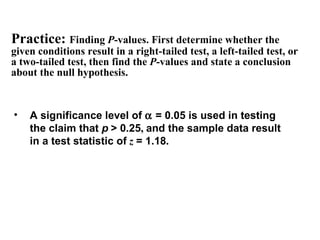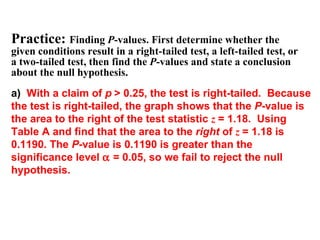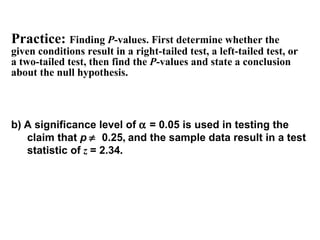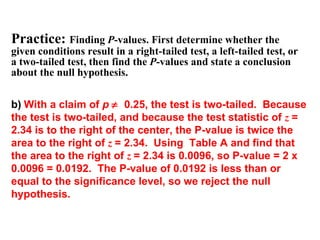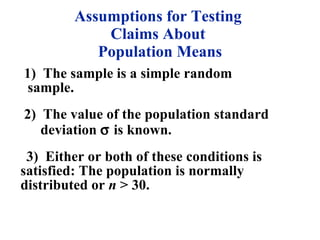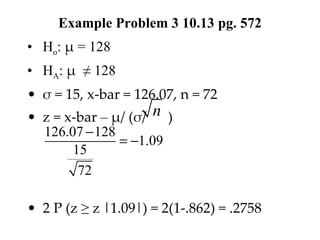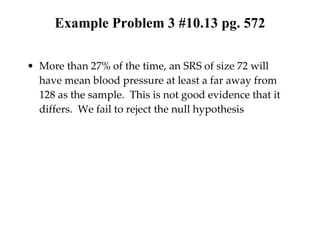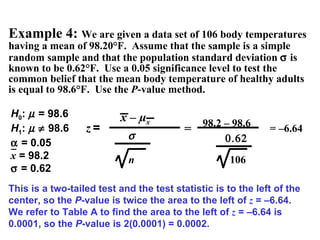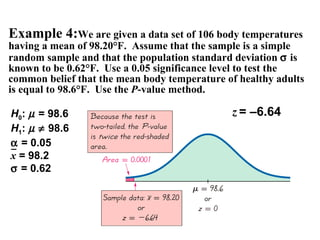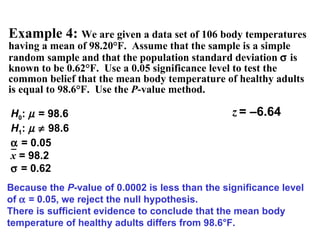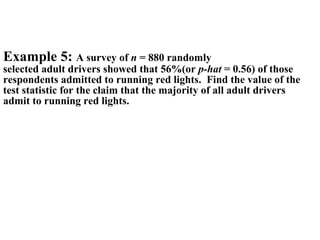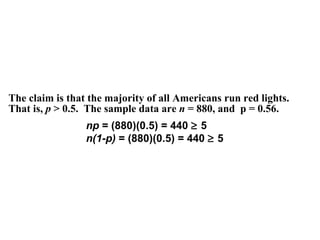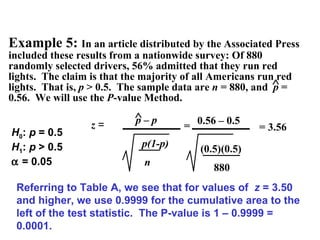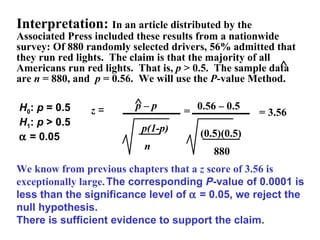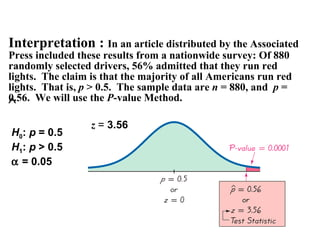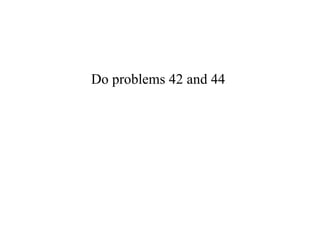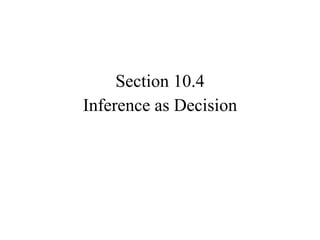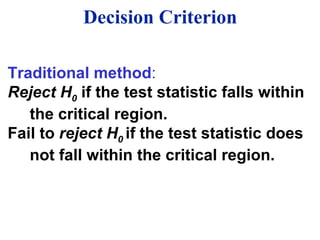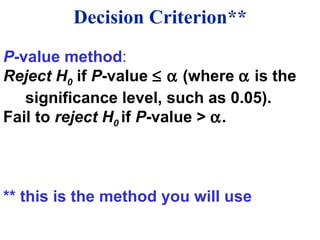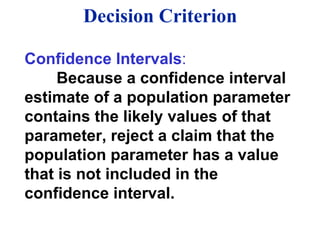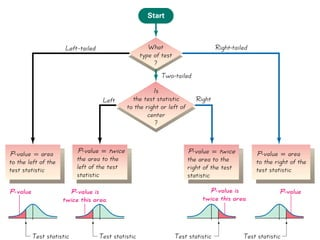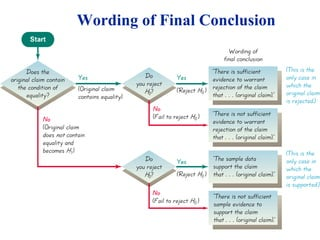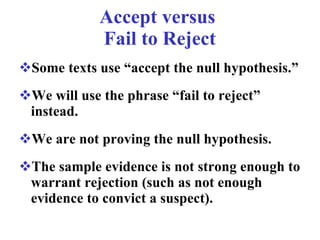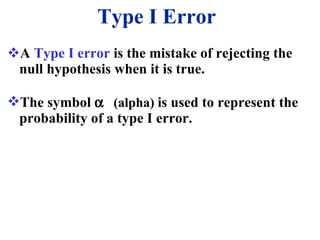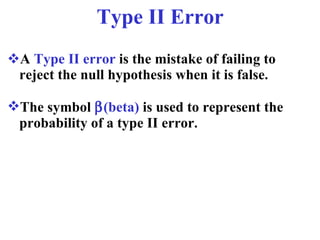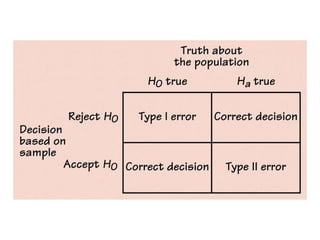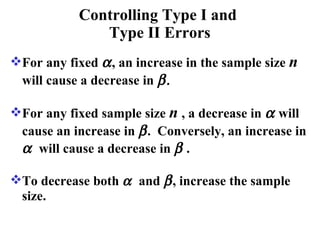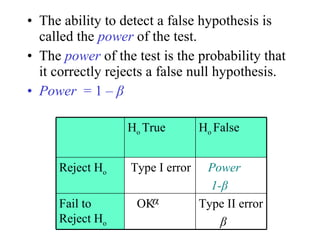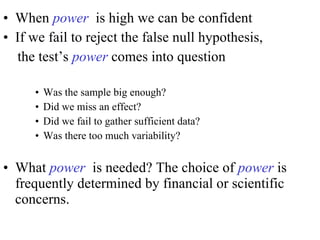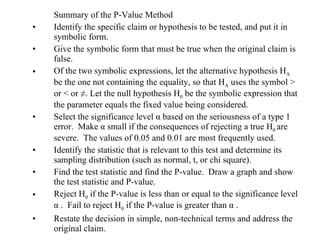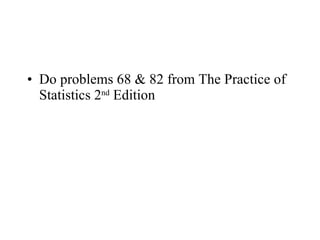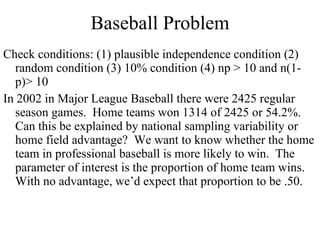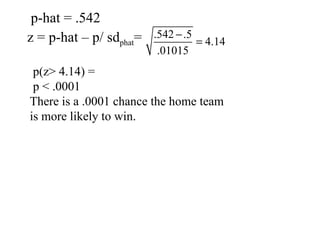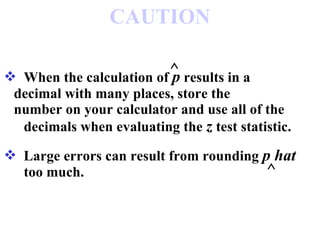1 de 77

### Chapter10 Revised

• 1. Chapter 10 Introduction to Inference Section 10.1 Estimating with Confidence
• 2. Twenty five samples from the same population gave these 95% confidence intervals, 95% of all samples give an interval that contains the population mean u.
• 3. In 95% of all samples, x-bar lies within ± 9 of the unknown population mean µ. Also µ lies within ± 9 of x-bar of the samples.
• 4. To say that x-bar is a 95% confidence interval for the population mean µ is to say that in repeated samples, 95% of these intervals capture the µ
• 5. The central probability 0.8 under a standard normal curve lies between -1.28 and + 1.28. That is, there is area .1 to the left and to the right of the curve.
• 6. The central probability C under a standard normal curve lies between –z* and + z* has area (1-C)/2 to its right under the curve, we call it the upper (1-C)/2 critical value.
• 7.
• 8.
• 9.
• 10.
• 11. Confidence Level C Single Tail Area (1-C)/2 Critical z value 90% .05 1.645 95% .025 1.960 99% .005 2.576
• 12.
• 13. Confidence Interval for p Single Proportion Confidence Interval for µ Single Mean ( σ known) Conditions Conditions If an SRS of size n is chosen from a population with unknown proportion p, then a level C Confidence Interval for p is: If an SRS of size n is chosen from a population with unknown proportion, µ then a level C Confidence Interval for µ is: p-hat ± z critical value ( ((p(1-p)/ n) X-bar ± z critical value ( σ /
• 14.
• 15.
• 16.
• 17.
• 18.
• 19.
• 20.
• 21.
• 22. Tests of Significance: We have learned that Confidence Intervals can be used to estimate a parameter. Often in statistics we want to use sample data to determine whether or not a claim or hypothesis about a parameter is plausible. A test of significance is a procedure in which we can use sample data to test such a claim. We will focus on tests about a population mean μ and proportions p.
• 23. Note about Identifying H 0 and H A
• 24.
• 25.
• 26.
• 27.
• 28. c) The standard deviation of IQ scores of actors is equal to 15. Practice : Identify the Null and Alternative Hypothesis. Express the corresponding null and alternative hypotheses in symbolic form.
• 29.
• 30.
• 31.
• 32.
• 33.
• 34.
• 35.
• 36. To determine the significance at a set level, alpha, one needs to calculate the P-value for the observed statistic. Careful consideration of the test statistic, z, can also be used to determine significance:
• 37. P -Value The P -value (or p -value or probability value ) is the probability of getting a value of the test statistic that is at least as extreme as the one representing the sample data, assuming that the null hypothesis is true. The null hypothesis is rejected if the P -value is very small, such as 0.05 or less.
• 38.
• 39.
• 40.
• 41.
• 42.
• 43. Critical Region The critical region (or rejection region ) is the set of all values of the test statistic that cause us to reject the null hypothesis.
• 44.
• 45. Practice: Finding P -values. First determine whether the given conditions result in a right-tailed test, a left-tailed test, or a two-tailed test, then find the P -values and state a conclusion about the null hypothesis. a) With a claim of p > 0.25, the test is right-tailed. Because the test is right-tailed, the graph shows that the P -value is the area to the right of the test statistic z = 1.18. Using Table A and find that the area to the right of z = 1.18 is 0.1190. The P -value is 0.1190 is greater than the significance level  = 0.05, so we fail to reject the null hypothesis.
• 46. Practice: Finding P -values. First determine whether the given conditions result in a right-tailed test, a left-tailed test, or a two-tailed test, then find the P -values and state a conclusion about the null hypothesis. b) A significance level of  = 0.05 is used in testing the claim that p  0.25 , and the sample data result in a test statistic of z = 2.34 .
• 47. Practice: Finding P -values. First determine whether the given conditions result in a right-tailed test, a left-tailed test, or a two-tailed test, then find the P -values and state a conclusion about the null hypothesis. b) With a claim of p  0.25, the test is two-tailed. Because the test is two-tailed, and because the test statistic of z = 2.34 is to the right of the center, the P-value is twice the area to the right of z = 2.34. Using Table A and find that the area to the right of z = 2.34 is 0.0096, so P-value = 2 x 0.0096 = 0.0192. The P-value of 0.0192 is less than or equal to the significance level, so we reject the null hypothesis.
• 48.
• 49.
• 50.
• 51. Example 4: We are given a data set of 106 body temperatures having a mean of 98.20°F. Assume that the sample is a simple random sample and that the population standard deviation  is known to be 0.62°F. Use a 0.05 significance level to test the common belief that the mean body temperature of healthy adults is equal to 98.6°F. Use the P -value method. This is a two-tailed test and the test statistic is to the left of the center, so the P -value is twice the area to the left of z = –6.64. We refer to Table A to find the area to the left of z = –6.64 is 0.0001, so the P -value is 2(0.0001) = 0.0002. = z = x – µ x  n 98.2 – 98.6 = –6.64  106 H 0 :  = 98.6 H 1 :   98.6  = 0.05 x = 98.2  = 0.62
• 52. Example 4: We are given a data set of 106 body temperatures having a mean of 98.20°F. Assume that the sample is a simple random sample and that the population standard deviation  is known to be 0.62°F. Use a 0.05 significance level to test the common belief that the mean body temperature of healthy adults is equal to 98.6°F. Use the P -value method. z = –6.64 H 0 :  = 98.6 H 1 :   98.6  = 0.05 x = 98.2  = 0.62
• 53. Example 4: We are given a data set of 106 body temperatures having a mean of 98.20°F. Assume that the sample is a simple random sample and that the population standard deviation  is known to be 0.62°F. Use a 0.05 significance level to test the common belief that the mean body temperature of healthy adults is equal to 98.6°F. Use the P -value method. z = –6.64 Because the P -value of 0.0002 is less than the significance level of  = 0.05, we reject the null hypothesis. There is sufficient evidence to conclude that the mean body temperature of healthy adults differs from 98.6°F. H 0 :  = 98.6 H 1 :   98.6  = 0.05 x = 98.2  = 0.62
• 54. Example 5: A survey of n = 880 randomly selected adult drivers showed that 56%(or p-hat = 0.56) of those respondents admitted to running red lights. Find the value of the test statistic for the claim that the majority of all adult drivers admit to running red lights.
• 55. The claim is that the majority of all Americans run red lights. That is, p > 0.5. The sample data are n = 880, and p = 0.56. np = (880)(0.5) = 440  5 n(1-p) = (880)(0.5) = 440  5
• 56. Example 5: In an article distributed by the Associated Press included these results from a nationwide survey: Of 880 randomly selected drivers, 56% admitted that they run red lights. The claim is that the majority of all Americans run red lights. That is, p > 0.5. The sample data are n = 880, and p = 0.56. We will use the P -value Method.  H 0 : p = 0.5 H 1 : p > 0.5  = 0.05 Referring to Table A, we see that for values of z = 3.50 and higher, we use 0.9999 for the cumulative area to the left of the test statistic. The P-value is 1 – 0.9999 = 0.0001. p(1-p) n p – p z =  0.56 – 0.5 (0.5)(0.5) 880 = = 3.56
• 57. Interpretation: In an article distributed by the Associated Press included these results from a nationwide survey: Of 880 randomly selected drivers, 56% admitted that they run red lights. The claim is that the majority of all Americans run red lights. That is, p > 0.5. The sample data are n = 880, and p = 0.56. We will use the P -value Method.  H 0 : p = 0.5 H 1 : p > 0.5  = 0.05 We know from previous chapters that a z score of 3.56 is exceptionally large. The corresponding P -value of 0.0001 is less than the significance level of  = 0.05, we reject the null hypothesis. There is sufficient evidence to support the claim. p(1-p) n p – p z =  0.56 – 0.5 (0.5)(0.5) 880 = = 3.56
• 58. Interpretation : In an article distributed by the Associated Press included these results from a nationwide survey: Of 880 randomly selected drivers, 56% admitted that they run red lights. The claim is that the majority of all Americans run red lights. That is, p > 0.5. The sample data are n = 880, and p = 0.56. We will use the P -value Method.  H 0 : p = 0.5 H 1 : p > 0.5  = 0.05 z = 3.56
• 59.
• 60.
• 61. Traditional method : Reject H 0 if the test statistic falls within the critical region. Fail to reject H 0 if the test statistic does not fall within the critical region. Decision Criterion
• 62. P -value method : Reject H 0 if P -value   (where  is the significance level, such as 0.05). Fail to reject H 0 if P -value >  . ** this is the method you will use Decision Criterion**
• 63. Decision Criterion Confidence Intervals : Because a confidence interval estimate of a population parameter contains the likely values of that parameter, reject a claim that the population parameter has a value that is not included in the confidence interval.
• 64.
• 65. Wording of Final Conclusion
• 66.
• 67.
• 68.
• 69.
• 70.
• 71.
• 72.
• 73.
• 74.
• 75.
• 76.
• 77.

### Notas do Editor

1. page 374 of text The term ‘accept’ is somewhat misleading, implying incorrectly that the null has been proven. The phrase ‘fail to reject’ represents the result more correctly.
2. Example on page 375 of text
3. page 377 in text